# Introduction

My class notes are compiled around the text book for the course:

• Herbert Schildt, Java: A Beginner’s Guide, 6th Ed., Oracle Press, 2014.

These notes are ordered based on my lectures, and video hyperlinks are provided below. The flow might not be ideal as a reading material.

• Install Java Development Kit (JDK)
• Test Java
• (Mac/Linux) Open the terminal. Type `java -version` and hit enter.
• (Windows) Start > Run > cmd. Type `java -version` and hit enter.
• Suggested Text Editors: SublimeText, Visual Studio Code, Atom, Emacs, Vim, NotePad++
• Suggested IDEs: Eclipse, IntelliJ, Netbeans
• If you are a novice Windows user, who wants to keep things simple, then only install Eclipse and use Notepad as your text editor for now.
• If you would like to be more involved in coding, then install SublimeText and Visual Studio Code alongside Eclipse.
• Some requirements
• Basic Java usage

## Hello World

• IDEs are not particularly useful while learning the syntax (as they tend to assist quite a bit). Instead, you are recommended to use a simple text editor for the first few weeks. Later, an IDE would be indispensable.
• For comments: Use `//` for single line, `/* text...*/` for multiple lines. It is important to document the flow of code with comments.

• Java syntax for the class with the main method:
``````class Classname {
public static void main (String[] args) {
//code block here
}
}
``````
• In the line that defines the main method, starting with public static…, inside the parenthesis, both `(String[] args)` and `(String args[])` would work. However, first one is technically more accurate as we will see in the definition of arrays later.
• Class name and file name must be same and extension must be .java
• javac compiles the java file:
``````javac Classname.java
``````
• javac produces executable Classname.class

# Fundamentals

## Numerical Operations

• We can define variables in 2 different ways:
``````Variabletype variableName;
variableName = somevalue;
// or alternatively
Variabletype variableName = somevalue;
``````
• Concatenation
``````System.out.println("string " + variable) // outputs string variable
System.out.println("string " + variable1 + variable2) // outputs string variable1variable2
System.out.println("string " + (variable1 + variable2)) // outputs string (sum of variable1 and variable2)
``````

## Data Types and Operators

• Primitive Data Types:Source: textbook, page 33

• integer / integer = integer. That means
``````int a,b;
a = 5;
b = 2;
System.out.println(a/b); // this will print 2, not 2.5
``````
• This might be the intended use, i.e., how many denominators are there in the nominator, ignoring the remainder. But if we need the mathematical result with the fractional part (as a double), how do we fix this issue?
• Define either one of the integers as double.
• Or convert either one of the integers to double: (double) var1/var2.
• Note that (double)(var1/var2) would not work, as that would do the conversion post integer division. Likewise, in the above example, if we tried `double c = a/b`, that would not have worked either. Variable c would be 2.0, as the right hand side is calculated first as 2.
• Do not rely on the equality of double variables. Upon a set of operations on two double variables, which should mathematically lead to the same result, it is very likely that the variables are not equal due to computer precision and the way these doubles are stored in memory.

# Program Control Statements

## For Loops

• Syntax of a for loop:
``````for (int -variablename- = -startingpoint-; -variablename- -checkingcondition- -boundvalue-; how -variablename- will be itearated) {
// code block here
}
``````
•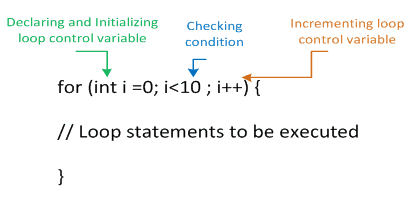Source: w3schools
• Initialization must be done before the scope of for. Others can be manipulated in the scope of for.
• Arithmetic Operations: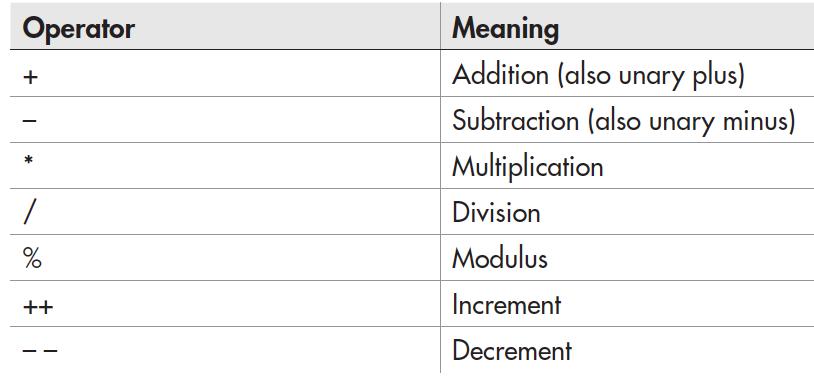Source: textbook, page 46
• Break kicks you out of the inner-most for or while loop.

## If Statements

• The general structure of an if-else statement is as follows: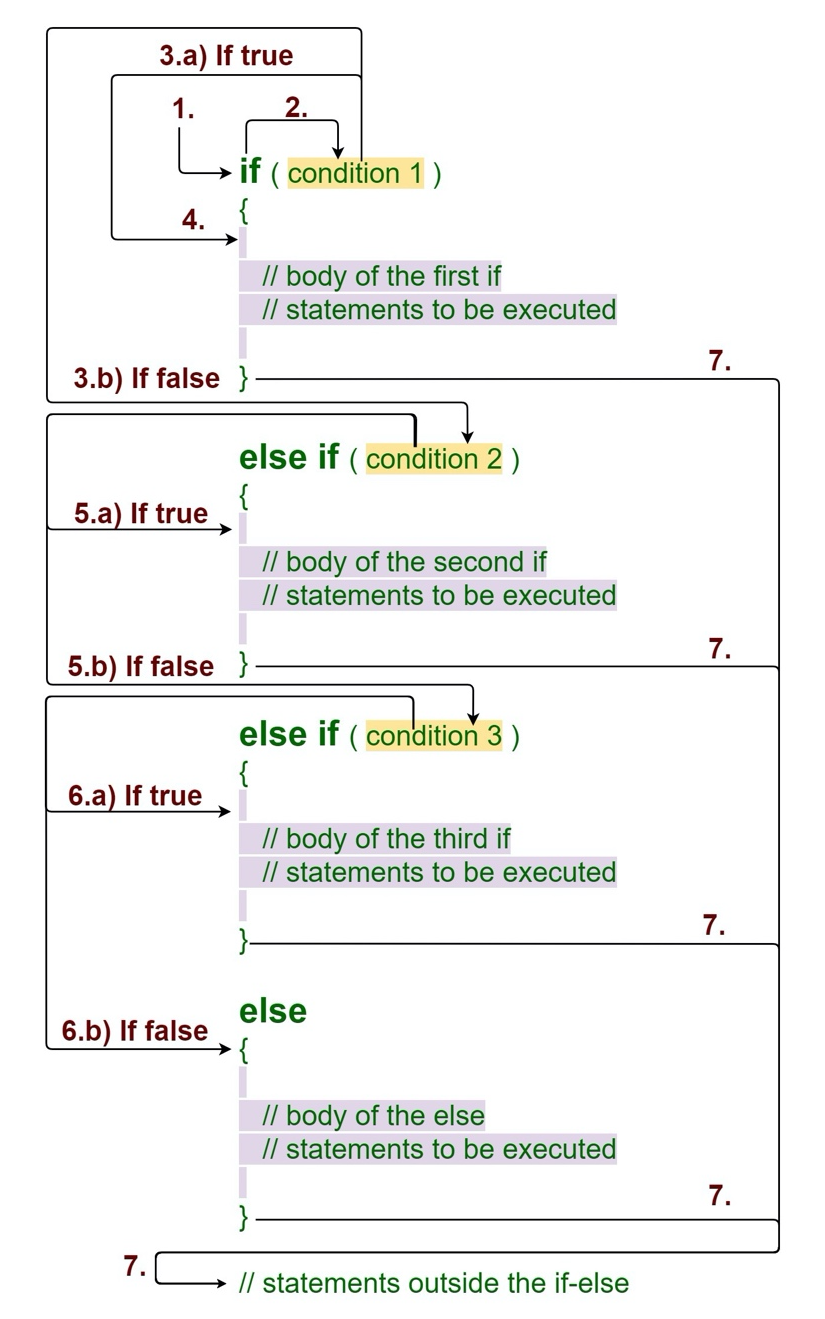Source: geeksforgeeks

• Curly braces provide a code block to be run without any disruptions. If there are no curly braces enclosing the body of a for loop or if statement, a single line is assumed to be the body.
• Give meaningful names to your variables. Technically it doesn’t matter, but it is a common practice to name them wisely for easier verification and maintainability of the code.
• Relational operators
• == : equal to
• != : not equal to
• < : Less than
• <= : Less than or equal to
• >: Greater than
• >= : Greater than or equal to
• Logical operators
• & : AND
• | : OR
• ^ : XOR (exclusive OR)
• || : Short-circuit OR
• && : Short-circuit AND
• ! : NOTDownload Files

## Questions

1. Write a Java code that prints all the numbers between 0 and 1500 that are divisible by 7 but not by 3 on the screen.

2. Write a Java code that prints sum of digit values for the numbers between 350 and 2570 that are not divisible by 7. For instance, sum of digit values of 375 is 3+7+5=15.

3.Write a for loop that prints the factorial of an integer on the screen. So the for loop should follow, for instance `int number=4;` and write `4!=24` on the screen.

4. Without compiling/running, predict what the following codes would output on the screen. If you think it would give an error during runtime or compilation, indicate the reason for the error and how it can be fixed.

``````boolean a = true;
boolean b = false;
if(a && !b)
System.out.print("Hello");
b = true;
if(a == false || b == false)
System.out.println("World");
if(b)
System.out.println("!");
``````
``````for (int index = 0; index < 3; index++) {
System.out.println("Welcome");
break;
}
``````
``````for(int i = 0; i <= 5; i++) {
System.out.println("i = " + i );
}
System.out.println("i after the loop = " + 1 );
``````
``````int value1 = 1;
int value2 = 2;
if(value1 == value2)
System.out.println("value1 == value2");
if(value1 != value2)
System.out.println("value1 != value2");
if(value1 > value2)System.out.println("value1 > value2");
if(value1 < value2)
System.out.println("value1 < value2");
if(value1 <= value2)
System.out.println(value1 <= value2);
``````
``````int s = 3;
int x = 7;
int y = 8;
int z = 11;
s++;
y--;
System.out.println("Output: " + (z + x + s) + " and "+ y);
``````
``````System.out.println("Hello");
System.out.print("World");
System.out.println("!");
``````
``````int result = 1 + 2;
System.out.println("1 + 2 = " + result);
int original_result = result;

result = result - 1;
System.out.println(original_result + " - 1 = " + result);
original_result = result;

result = result* 2;
System.out.println(original_result + "* 2 = " + result);
original_result = result;

result = result / 2;
System.out.println(original_result + " / 2 = " + result);
original_result = result;

result = result + 8;
System.out.println(original_result + " + 8 = " + result);
original_result = result;

result = result % 7;
System.out.println(original_result + " % 7 = " + result);
``````
``````for(int i=1; i<65; i++) {
if(i%3==0) {
if(i%2==0 && i%5!=0)
System.out.println(i);
}
}
``````

## Primitive Data Types

• Math library of Java: You can use for more complex mathematical operations such as sine, cosine, logarithm etc.
• For now, you can memorize some use cases we discussed but it’s good to know why/how we use the periods in that syntax.

• Popular Example:Source: Smartherd
• Function (Method): A block of code that performs a specific task. There are several built-in functions, e.g., math.sqrt(). You can design your own functions hopefully in 3 weeks :)

• You can increment/decrement chars similar to an integer. You can even initialize them as integers.
• Every char has an ASCII number (they are basically integers, see figure given below). You access any char with the associated numeric value.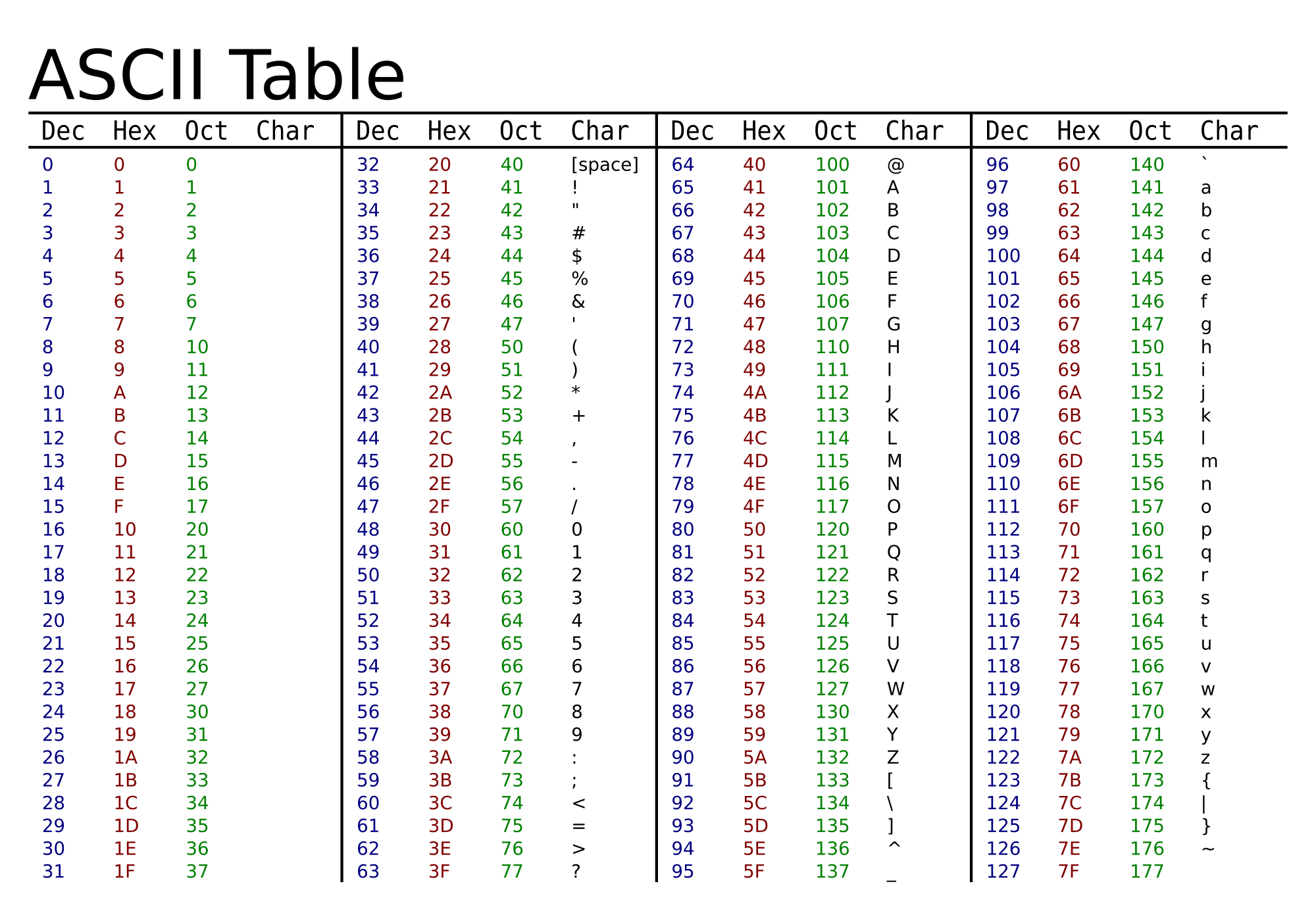Source: Harvard CS50 Course (you may want to review this course)
• Long to integer conversion: Since integer has smaller domain, compiler gives error. Same error on integer to char conversion because there are fewer characters than integers.
• Compiler converts any validation statement true or false (e.g., `(10>9)`) to boolean.

• Java syntax for the class with the main method:
``````class -classname- {
public static void main (String[] args) {
//code block here
}
}
``````

## Scope of Variables

• A code block is statements written between curly braces.
• Scope is the region where defined variables are usable.
• The scope of a variable depends on where you define a variable. It starts right after the point of declaration, goes until the end of the block.
• A variable is not usable outside (i.e., before/after) its scope.
• In the scope of a variable, you cannot define another variable with the same name.
• You cannot refer to a variable before its declaration.
• Scope Example: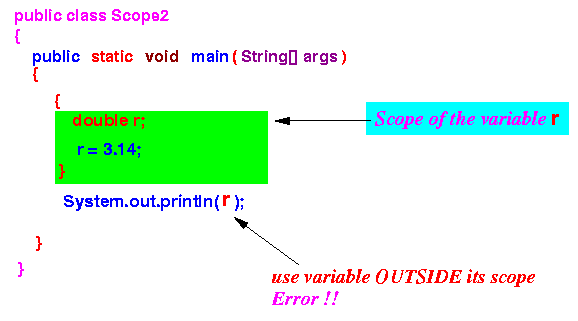Source: Emory University
• Initialization error: When you declare a variable and use it possibly without initialization.
• To be on the safe side, initialize variables when/after you declare.

## Input Mechanisms

• User input is dynamically changing in real life.
• Getting input from the user (once): `System.in.read()`
• Casting: changing data type into a different data type. Usage: new data type in parenthesis, e.g., (double)
• Some escape characters: `\n` : new line `\t` : tab
• Using “java classname inputs” command is another (and much more flexible) option to receive user input.
• Arrays - A quick preview:
• Consider arrays as vectors
• Syntax: datatype[] arrayname (we will cover arrays in detail later)
• Index (address) of an array starts with 0
• You can access any element of an array by using its index. Example: arrayname (returns 6th element of array)
• When we have our usual main method line, args array is automatically created for you. It’s actually what’s input to the main method. We will cover these in detail while discussion methods.
• Double.parseDouble(data): casts data into a double
• Integer.parseInt(data): casts data into an integer
• We have to make sure these types can be casted. Not all casting is possible or makes sense. But a string of say “6” can definitely be converted to an integer.
• Remember some casting is easier as mentioned on the third bullet above; (double) would convert an int to a double.

We have covered some topics from future chapters too but make sure that you read up to Pg.69 in the textbook.Download Files

## Questions

1. Write a Java code that prints all the letters in the alphabet in lowercase (char decimals 97-122) in a single line without any space in between.
2. Write a Java code that prints all the letters in the alphabet in lowercase (5 letters per line) without any space in between.
3. Write a Java code that prints all the letters in the alphabet in lowercase (move to the next line if e or t is to be printed next) with one space in between.
4. We can cast a long into an int without any information loss. True or False?
5.Write a Java code that prints the prime numbers between 350 and 2570.
6. Get several integers from the user with the java call and print the summation. With the java call implies your code should be implemented as in the following example:
``````java sum 3 5 -13 8 13
sum of given numbers is 16
``````
7.Write a code that reports the sum of odd numbers in a given sequence. The numbers are given with the java call. Let the name of the program be sumOdd so we should be able to call it using the command `java sumOdd -numbers-`.
You can ignore negative numbers.
``````java sumOdd 66 3 5 44 7 3 8 -5 9 22 6 -4 1250
sum of odd numbers in this sequence is 27
``````
8. Write a code that reports the second largest number in a given sequence. The numbers are given with the java call. Let the name of the program be secMax so we should be able to call it using the command `java secMax -numbers-`.
Note that max and second max might be the same.
``````java secMax 3 5 7 8 9 22 -5 -3 6 0
second max is 9
``````
9.Write a code that reports the second smallest “value” in a given sequence. The numbers are given with the java call. Let the name of the program be secMin so we should be able to call it using the command `java secMin -numbers-`.
Note that the min and second min values cannot be the same.
``````java secMin 3 5 28 28 9 22 -5 -3 6 0
second min is -3
``````
10. Print the equivalent integer for a user-given character using casting.
11. Get an integer value from the user with the java call and
• print if it is an odd or even number,
• print if it is a prime number or not,
• print as many *’s.
12. Get two integer values from the user (ordered as a and b) with the java call and print the b-th power of a.

## Switch Statement

• Java syntax for the switch:
``````switch(expression) {
case x:
//code block here
break; //break is optional but very common if you want to do one code block for each case
case y:
//code block here
break;
default: //default is optional
//code block here
break;
}
``````
• Switch:Source: tutorialspoint

• If you do not use break, it executes every statement till the break statement. Break is optional.
• Default can be interpreted as else, i.e., when none of the above cases applies (or they are taken and no break is included). Default is optional.
• enum is special class that represents a group of constants. To create an enum, use the enum keyword instead of class and separate the constants with a comma.
``````enum -enumname- {
-CONSTANT(1)- , -CONSTANT(2)- , ... , -CONSTANT(N)-
}
``````
• Please investigate the use of `->` in switch statements, instead of `:`. That automatically breaks the associated code block, making it a very convenient and frequently-used alternative.

## While, Break and Continue

• While syntax:
``````while (statement) {
// ''while'' statement is true, executes the code block
}
``````
• Do-While syntax:
``````do {
//executes the code
} while(statement)  //loop will continue ''while'' statement is true
``````
• Difference between while and do-while:Source: Anonymous
• Break kicks you out of the inner loop.
• Break: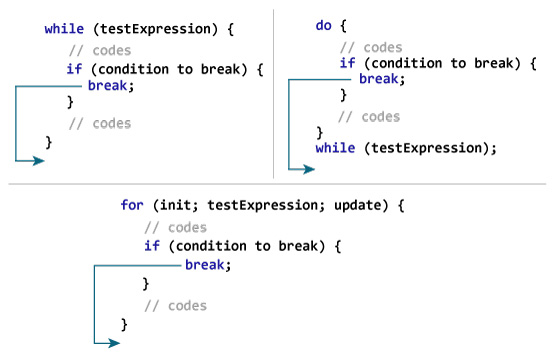Source: programiz
• You can define code blocks and use the break (break out of defined scope):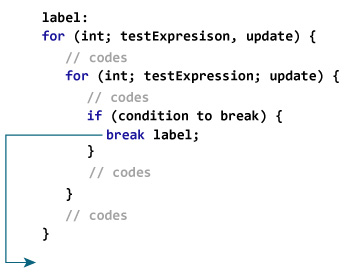Source: programiz
• Continue: If condition is true, ‘‘continue’’ to the inner loop. (It will skip the rest of the code block within the loop).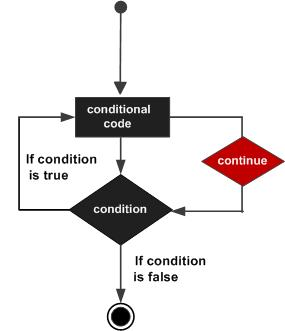Source: programiz

## Arrays

• Array can be used to store a vector (one-dimensional array) or a matrix (multi-dimensional array) in mathematical operations.

• Syntax(one-dimensional):

``````-data_type-[ ] -array_name- = new int [-length_of_array-];
-array_name- [-index-] = -value-;  //Assign values
``````

Example:

``````int[] exampleArray = new int;
exampleArray = 2 // First element assigned to 0
``````
• Syntax (multi-dimensional with fixed length arrays):
``````-data_type-[ ][ ] -arrayname- = new int [-length_of_array-][-length_of_array-];
-array_name- [-index-] [-index-] = -value-;  //Assign values
``````
• Since the multi-dimensional array is the array of an array, you can specify the length of the arrays separately:
``````-data_type-[ ][ ] -array_name- = new int [-length_of_array-][ ];
-array_name- [-index-] = new int [-length_of_array- ]; //Creates array for given index.
``````
• Alternative definition:
``````-data_type-[ ] -array_name- = {-value(0)-, -value(1)- , ... , -value(n)- } //Creates array length of (n+1)
``````
• Initialize arrays when you declare.
• Array indexing:Source: GeeksforGeeks
• Array (a multi-dimensional example with same length arrays)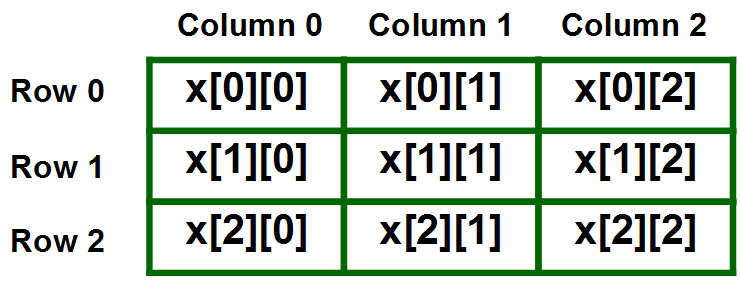Source: GeeksforGeeks
• Pseudocode: Half code half definition. A code-like explanation of an algorithm, without worrying about the syntax. All that matters is the logic and flow of algorithm and clarity.

## Enhanced For Loops

• Super useful especially in object oriented programming.
• When you master the use of enhanced for loop, you will be able to write codes that make sense to everyone that needs to maintain the code.Download Files

## Questions

1. Write a code that utilizes a switch statement and array of days to report the day of the week, given a number with the java call. Let the name of the program be dayofWeek so we should be able to call it using the command (java dayofWeek -number-). Your array should contain all days of the week. You can include the following line in your code:
``````String[] days = {"Monday", "Tuesday", "Wednesday", "Thursday", "Friday", "Saturday", "Sunday"};
``````

Here are some examples for clarification:

``````java dayofWeek 3
That day is Wednesday
java dayofWeek 14
Enter a number between 1 and 7
java dayofWeek -3
Enter a number between 1 and 7
``````
2. Write a code that utilizes a switch statement and reports the month, given a number with the java call. Let the name of the program be month so we should be able to call it using the command (java month -number-). Here are some examples for clarification:
``````java month 3
That month is March
java month -3
Enter a number between 1 and 12
``````
3.Write a code that finds the median of given integers. That is, when ordered, the number in the middle; or if there are two numbers in the middle, their average. Here are some examples:
``````java median 3 5 8
The median of 3 5 8 is 5
java median 8 5 4 -10
The median of 8 5 4 -10 is 4.5
``````

Hint: First depending on the size of the given array, decide if there would be a number in the middle (odd sized arrays) or not (even sized arrays). Use another array (say flag) and iteratively compute max and min of the given numbers if they are unflagged - initially all are unflagged so start with all false (if array of booleans) or zero (if array of integers) on the flag array. Flag the numbers chosen, that is set maximum’s and minimum’s associated flags to true or one. Repeat finding this min/max among unflagged for the required number of steps, and you will be left with one or two unflagged numbers. Use that information to compute the median.

# Classes, Objects, and Methods

## Primitive vs. Class Types

• Summary## Functions

• A function is a block of code that perform certain actions.
• General Syntax:Source: Princeton
• There can be multiple argument variables (i.e., input parameters). You have to define types of variables. When you call your function, you must give parameters to your function in a same order.
• When you give a name to your function, be careful. Don’t use reserved keywords and give reasonable names.
• Two types of function: Functions that return a value (e.g., integer array, double, String etc.) or void type functions(i.e., methods which do not return a value). You must declare it when you define your function.
• Usage (function calling):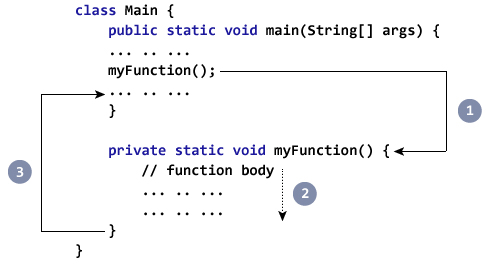Source: Programiz
• Don’t manipulate original data (i.e., input parameters) in your functions. You may want to do it for a purpose but if you don’t have one, use copy of parameter. Otherwise, if the input parameter is a reference or class type, note that you’re changing it elsewhere too.

## Objects

• A class is a template that defines the form of an object. It specifies both the data and the code that will operate on that data.
• Syntax :
``````class -class_name- {
//define member variable(s)
//define member function(s)
//define constructor(s) (if you don't define any constructor, default constructor will be utilized.)
}
``````
• In main method, you can create objects:
``````-class_name- -object_name- = new -class_name-();
// e.g.,
Person john = new Person();
``````
• Accessing the member functions:
``````-object_name- . -function_name- (-parameter(s)-) ;
// e.g.,
john.calculateBMI();
``````
• Accessing the member variables:
``````-object_name- . -variable_name- ;  //returns value of member variable.
// e.g.,
john.bmi;
``````
• Java uses a class specification to construct objects. Objects are instances of a class.
• A class is essentially a set of plans that specify how to build an object.
• A class is a logical abstraction. It is not until an object of that class has been created that a physical representation of that class exists in memory.
• Methods and variables that constitute a class are called members of the class.
• The new operator dynamically allocates (that is, allocates at run time) memory for an object and returns a reference to it. This reference is, more or less, the address in memory of the object allocated by new. This reference is then stored in a variable. Thus, in Java, all class objects must be dynamically allocated.Download Files

## Questions

1. Given two arrays (say array1 and array2) of size 3 (that is you assume int[] array1 = new int; and int[] array2 = new int; are already in place), write 4 more statements (no loops etc.) that ensures values on both arrays are [0,1,2].
2. Write a method that prints the factorial of an integer on the screen. So when called, for instance as `factorial(4);`it should write `4!=24` on the screen.
3. Define a method that takes a number as input and returns if it is prime or not.
4. Use switch case in a program that will provide the associated letter grade for a point grade, i.e., A if it is in [95-100], C in [65,70), D in [50,55) etc. One should be able to call it using the command `java letterGrade -number-`
5. Write a method that takes an array of integer values and returns the reverse of that.
6.Write a method that prints the common elements between two arrays of strings.
7. Write a method that returns the middle character of a string.
8.Write a method that returns the number of even integers in a given array.
9. Write a method that returns the difference between the largest and smallest values in a given double array.
10.Given an array of integers, write a method that returns true if a number appears “exactly” twice, and returns false otherwise. Optional: Print out the locations and the number.
11. Clump is defined as a series of 2 or more adjacent elements of the same value. Write a method that returns the number of clumps in a given array.
12. Given an array, write a method that returns true if there is a place to split the array so that the sum of the numbers on one side is equal to the sum of the remaining numbers on the other side.
13. A nonnegative integer is called a palindrome if it reads forward and backward in the same way. For example, the numbers 5, 121, 3443, and 123454321 are palindromes. Write a method that takes as input a nonnegative integer and returns true if the number is a palindrome; otherwise, it returns false.

## Eclipse IDE and Code Conventions

• The reason why we used text editor so far is to have a good grasp of the Java syntax.
• In real life we mostly use IDEs for projects.
• We will use Eclipse as IDE in this course. However it might look slightly different depending on the version or OS. Understand the logic then google any issue you face first.
• First you have to create a java project
• src: where source files are usually located. You have to locate your .java files here. In general, you need to know where your projects are in your computer and how they are organized.
• Outline shows member functions, variables etc. in a class.
• You can check conventions from Oracle’s web-site: Oracle Naming Conventions
• Naming convention:

• Classes starts with upper-case (e.g., class TwoPeople)
• Functions and variables: lower-case first word, upper-case other words. (e.g., myVariable, runFast())
• Constants (i.e., data types that we never change) : upper-case letters (e.g., static final int MIN_WEIGHT=10.)
• Long names are fine (unless too long). Be crystal clear when you are naming.
• IDEs provide excellent features: auto-complete, warnings, suggestions etc.
• Debug: Super-powerful life saving mode.
• Break points can be interpreted as milestones. When you debug your code, you can define intervals with break points.
• Step into: Goes into the function.
• Step over: Immediately jumps into next line.
• From the expressions tab, you can query values, call functions etc. It depends on scope of the debug.
• Important: You can access the source codes, if need be, by right-clicking on the java file on the Eclipse Project Explorer pane (on the left by default) and choosing Show In > System Explorer.
• Important: If you need to pass args using Eclipse, rather than running from the terminal window, you need to edit the Run Configuration, or create new Run Configuration(s). You can either use the Run Configuration menu after right-clicking on the project on the Eclipse Project Explorer pane, or by choosing the little down arrow next to the run button. Under Run Configurations, you will notice an Arguments tab, where you can add args inside the Program Arguments text box.

## Constructors

• Initialization of an object:
``````-Classname- -objectname- = new -Classname(-constructor input-)
``````
• When we use default constructor, it creates objects with default values.
• Syntax (Constructor):
``````-Classname(-inputs-){
this.-variableName- = input   //declaring the value of variable
this.-variableName- = -functionName(input)- //you can call function inside the constructor and manipulate inputs.
}
``````
• Use `this` for avoiding confusions when dynamically changing variables for an object.
• When you create an object, inputs should be in same order and data-type with how you define the constructor. Example:
``````Person(int age, double weight, int height, boolean female){
// code block
}
Person erhun = new Person( 25(int age) , 75.3(double weight), 182(int height), false(boolean female) )
``````
• Constructors do not have a return type.
• (Recommended) Don’t do anything other than initialization of your object in constructors.

• Garbage Collection (deconstruction): Java automatically clean the memory for you. You do not need to worry about. However you can deconstruct any data type manually.

• Overloading means you can define either a constructor or a method multiple times with different type of inputs.

``````Person(int age, double weight, int height, boolean female){
// code block (first consturctor)
}

Person( ){
// code block (second consturctor)
}

//You can initialize your object in two ways:
Person erhun = new Person(25, 75.3, 182, false);
//OR
Person erhun = new Person();
``````
• You can also overload functions:
``````public double calculateBmi(){
// code block (first function)
}

public double calculateBmi(double height, int weight){
// code block (second function)
}
``````
• If you declare that two objects are equal, then you make their pointers equal (i.e., both objects point the exactly same location in memory). When you update one object, both will be updated.

## Access Modifiers

• The access modifiers in Java specifies the accessibility or scope of a field, method, constructor or class.
• When no access modifier is specified, default access modifier is default.
• Access modifiers and their limitations: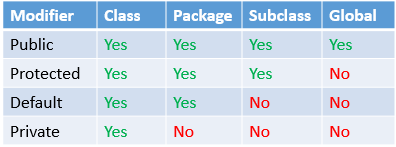Source: net-informations
• Getter/Setter functions: When you restrict the access of the variable for any scope, you can access the values with getter functions and update the values with setter functions. You can also specify the scope of getter and setter functions. Example:
``````public double getWeight(){
return this.weight; //returns the value of the variable
}

public void setWeight( double weight){
this.weight = weight; //updates the value of the variable
}
``````
• setWeight(-double weight-) function does exactly the same thing with updateWeight(-double weight-) function. You do not have to give a specific set or get names. However, we call them setter or getter by convention :)Download Files

## Questions

1. Write an Author class with following features:
• Instance variables:
• firstName for the author’s first name of type String.
• lastName for the author’s last name of type String.
• Constructor:
• public Author (String firstName, String lastName): A constructor with parameters, it creates the Author object by setting the two fields to the passed values.
• Instance methods:
• public void setFirstName (String firstName): Used to set the first name of author.
• public void setLastName (String lastName): Used to set the last name of author.
• public double getFirstName(): This method returns the first name of the author.
• public double getLastName(): This method returns the last name of the author.
2. Consider the Movie class below.
``````public class Movie {
private String title;
private int rating
}
``````

An instance of class Movie represents a film. This class has the following variables:

• title, which is a String representing the title of the movie
• rating, which is an integer representing the imdb rating of the movie
Write a constructor for the class Movie, which takes a String representing the title of the movie, and an int representing the rating as its arguments, and sets the respective class variables to these values.
3. Can a class have multiple constructors?
4. Can a class have multiple constructors with same number and types of inputs?
5. Create a Rectangle class that has the length and width attributes, and a constructor that takes two inputs and always assigns the smaller value to width and larger to length. Also include methods getArea() and getPerimeter(). Then, create two Rectangle objects and compare (print the values returned by the getter methods) their areas and perimeters.
6. Write a program that would print the information (name, year started, salary, address) for three employees by creating a class named ‘Employee’. There should be a row that indicates type of information and three rows for three employeees in spreadsheet format with tabs in between columns.
7.Write a RightTriangle class with two edge lengths stored as variables. Include a constructor that takes two values as input and sets these variables. Include the necessary code so that I can do the following from the main method.
``````RightTriangle rightTriangle = new RightTriangle(3,4);
double hyp = rightTriangle.hypotenuse(); // hyp gets value 5.0
double a = rightTriangle.area(); // a gets value 6.0
double p = rightTriangle.perimeter(); // p gets value 12.0
double s = rightTriangle.smallestAngle(2); // s gets value ~ 53.13 which is the 2nd smallest angle for the triangle.
double ss = rightTriangle.smallestAngle(1); // ss gets value ~ 36.87 which is the 1st smallest angle for the triangle.
``````

# Random Number Generators and Simulation

## Introduction to Martingale

• Martingale is a well-known gambling strategy.
• Martingale betting strategy: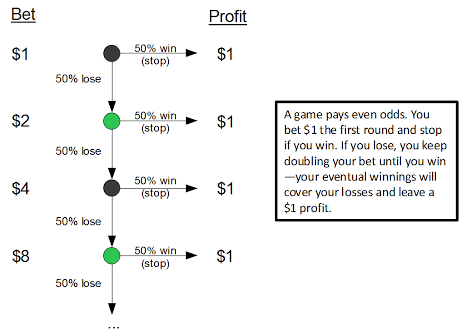Source: Anonymous
• Drawback of the martingale system is that bet amount rises exponentially (nobody has infinite wealth) and profit is low: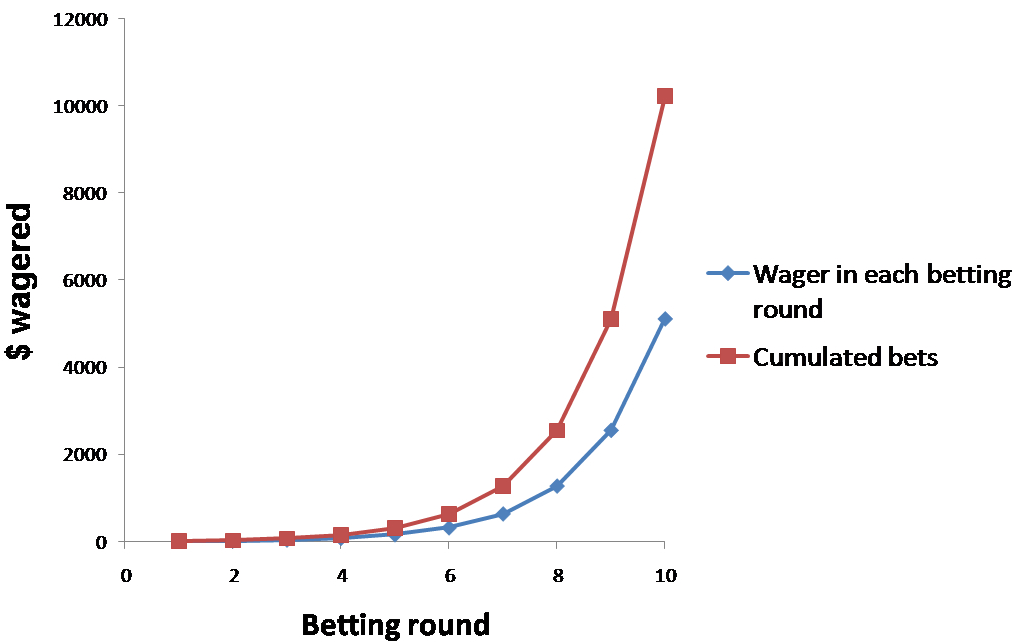Source: pokerbankrollblog
• Keep in mind: House always wins!

## ArrayList

• In arrays, we have to declare the size and it is fixed. What if we need to change the size dynamically?
• ArrayList allows you store your data in a vector without declaring the size and add/remove while vector size adapts these actions dynamically.
• Syntax (Constructor):
``````ArrayList<"datatype"> -arraylist_name- = new ArrayList<"datatype">();

``````
• ArrayList Functions:
• .add(-element-): Adds a new element to the end of the ArrayList; the return value is always true.
• .add(-index-, -element-) : Inserts a new element into the ArrayList before the position specified by index.
• .remove(-index-) : Removes the element at the specified position and returns that value.
• .remove(-element-) : Removes the first instance of element, if it appears; returns true if a match is found.
• .clear(): Removes all elements from the ArrayList.
• .size(): Returns the number of elements in the ArrayList.
• .get(-index-): Returns the object at the specified index.
• .set(-index-, -value-): Sets the element at the specified index to the new value and returns the old value.
• .indexOf(-value-): Returns the index of the first occurrence of the specified value, or -1 if it does not appear.
• .contains(-value-): Returns true if the ArrayList contains the specified value.
• .isEmpty(): Returns true if the ArrayList contains no elements.
• Note: Try to declare your variables as private inside the class as much as you can.

## Random Numbers and Outcomes

• Keep in mind, your functions can also return objects.
• Recall: Generalized syntax for return type functions:
``````-access_modifier- -return_type-  -function_name- (-input_parameters_with_types-){
// code block
return -return_variable-;
}
``````
• Random numbers make it possible to write programs that simulate random processes.
• Math.random(-integer_value-): generates double number in between [0,-integer_value-) Note: default [0,1)
• Is it possible to generate ‘‘true’’ random numbers? For those who are interested: True Random Numbers

## Seeds

• Seeds enable us to replicate a simulation. Whenever you use seed number, computer will generate same random numbers in the same order.
• To generate random numbers from a seed, you need a random object.
• Syntax:
``````import java.util.Random;
Random -name- = new Random();
-name-.setSeed(-seed_number-);
``````
• Some other functions:
``````-name-.nextInt(-integer_value-); //generates integer random number in between [0,-integer_value-) - default [0,1)
-name-.nextDouble(); //generates double random number in between [0,1)
``````Download Files

## Questions

1. Provide a class named die, that uses a Random object and has two methods: one that returns the outcome for a die roll, and one that returns how many given values are observed among a given number of rolls. I should be able to do the following:
`````` Random random = new Random();
Die die = new Die(random);
int outcome = die.roll(); // outcome becomes 1,2,3,4,5, or 6.
int count = die.experiment(5,10000) // count becomes how many 5's are observed among 10000 die rolls.
``````
2.Assume that there is a slot machine with 6 different outcomes; Tree, Apple, Star, Ball, Flower, Cat. There are three slots, and in each slot, all six possibilities are equally likely. If all three slots show the same outcome at the end of a round, you get back three times your bet. If two of the slots have the same outcome, you receive twice your bet. If none of the slots have the same output, you do not win anything. Each round should be played within a dedicated Java method (input and output for the method is up to you). The initial bet amount is \$5. Each time you win (by doubling or tripling), you double your next bet. Each time you lose, you bet the same amount as you did in the previous round. If you do not have enough money, you bet whatever you have (all-in). You will stop playing after 200 rounds, or when you are broke. The output should look like this:
``````Initial amount: 100
The slot machine was played 189 rounds. The bet was tripled 3 times, doubled 5 times, and lost 181 times. The final money you have is 0.
``````
3. In the above question, add the following information to the output:
``````Maximum amount won in a round was 20 by betting 10.
``````
4.Write a method that generates a phone number. The phone number must start with 05 and must have 11 digits total: 0 5** *** ** **. The numbers after 05 will be generated randomly one by one. Method should return the phone number as a string with the spacing described in the format 0 5** *** ** **.
5.Write a Java class called playRoulette that gets an integer input from the user between 1-36. Then 2 dice are rolled randomly and their output is printed on the screen. Print out whether the user made the right guess for the multiplication of the two dice values.
``````java playRoulette 24
The dice outcomes are 2 and 6.
You lose!
java playRoulette 28
Hey genius! You lost before the dice are rolled!
java playRoulette 15
The dice outcomes are 3 and 5.
You win!
java playRoulette 17
Hey genius! You lost before the dice are rolled!
``````
6. Write a method that generates an exponential random variable using the input as the rate. Your method should be implemented as:
``````double y = Exponential(5); // y takes the value of a randomly generated exponential random variable with rate 5
``````

## Knapsack ProblemMatiasEmilioDelgado

• Definition: Given weights and values of n items, put these items in a knapsack with capacity W to get the maximum total value in the knapsack.
• Formulation: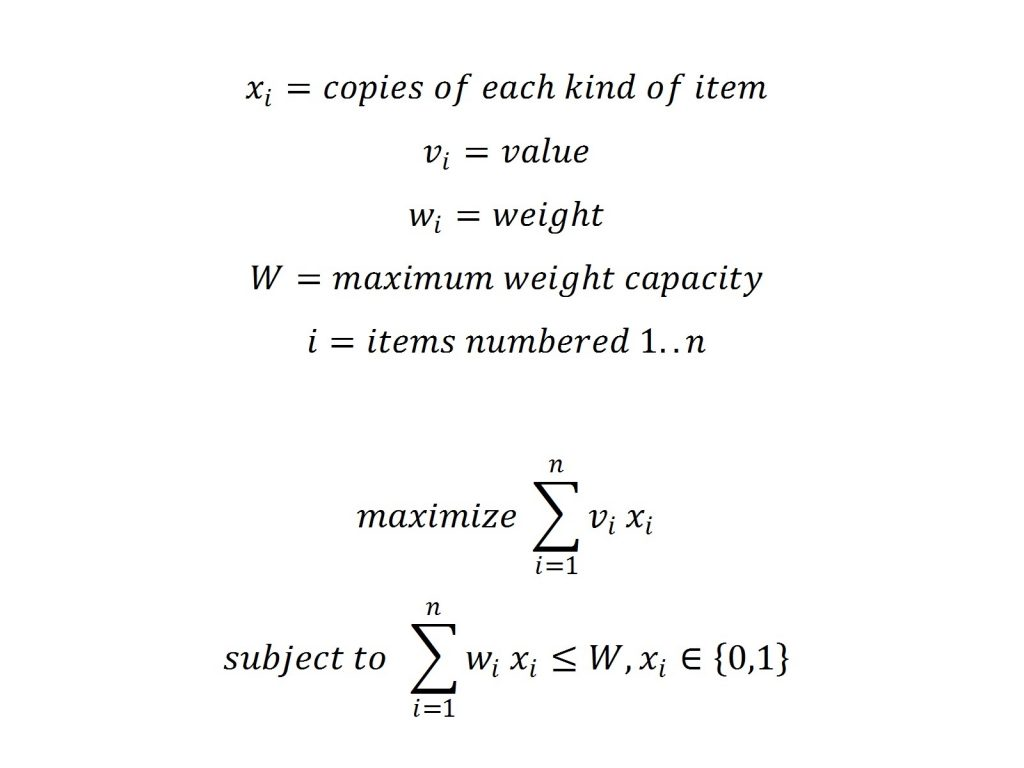Source: The Optimization Expert

• Combinatorial Optimization: Discrete (countable) number of feasible solutions.
• Combinatorial optimization problems are usually computational challenging!
• For more details for geeks about computational complexity, wikipedia page is a good start: Computational Complexity
• Also you can check the following lecture on complexity

• In real life, pulling data from excel or txt is a weak practice. Generally database and json format are used since they are more structured!
• What is database? Check Oracle web-page: What is Database?
• In this lecture, we will cover raw text file: We will use csv (comma-separated values) but reading from txt works the same way too.
• Recall: Generalized syntax for return type functions:
``````import java.io.FileReader;
// OR
//import java.io.* // imports everything in java.io!
try {
FileReader -filereader_name- = new FileReader("-data-");  // You will give path to the data. Full path is not needed if the file is in the same folder with the class file.
String[ ] -array_name- =  -line_name-.split("-split_char-"); // "split" "given line of string" into elements  according to given "split_char"
} catch (FileNotFoundException e) {
System.out.println(e); // If your data.csv file can not be found*
} catch (IOException e) {
System.out.println(e); //
}
``````

## Structuring the Code

• When you are dealing with complex structures, you should write your code in a “modular” way.
• A heuristic solution for the knapsack problem:
• Define value/weight for every item.
• Sort them in a descending order.
• Start from the first item (i.e., biggest in terms of value per weight)
• Include item if there is enough capacity, otherwise check the next item in sorted array.
• Heuristic approach: Although it can find a (perhaps good) solution, you cannot guarantee that the solution is optimal.
• Next week, we are going to solve the same problem with a commercial solver.

## Use of HashMapEyjolfurSverrisson

• Syntax:
``````HashMap <-datatypeForKey-,-datatypeForValue-> -hashmap_name- = new HashMap <-datatypeForKey-,-datatypeForValue->();
``````
• Hashmap Functions:
• .put(key, value) // add element to the HashMap
• .put(key, value) // replace element with the associated key (because keys are unique)
• .keySet() // returns all keys. Note that hashmap.keySet() can be iterated (i.e., in an enhanced for loop)
• .values() // returns all values. Note that, hashmap.values() can be iterated (i.e., in an enhanced for loop)
• .remove(key) // remove given key and associated value.
• .clear() // clear all key-value pairs of HashMap
• .size() // returns the size of the HashMap (number of key-value pairs)
• Notes:
• HashMap has unique keys. So no two keys can be the same.
• Keys are never primitive types, but class types (objects). It can be a predefined type such as Integer, or a type you created.
• Keep in mind that the key is actually a pointer. So it doesn’t really search the HashMap as you would search a dictionary one by one. It uses the pointer referring to the object and access the associated value using that pointer. That is why HashMap is an extremely efficient data structure in retrieval.
• What that pointer logic means in implementation is that if you create two objects with the exact same variable values, they are actually two different objects that can be two separate keys.
• While using HashMaps, iterate through the objects themselves and look for associated values.
• In case of HashMap of a HashMap, it is still a HashMap inside so the same properties hold, each key must be unique. Here is an example: distances between two cities. Suppose we create “City” objects. You can do HashMap<City,HashMap<City, Double>> distances, where the first key is origin city, and the latter is the destination city.
• If you only to distances.get(c) that would return a HashMap, where keys are all cities and values are distances from city c to each city.
• So from City c to City d you can compute the distance using distances.get(c).get(d).Download Files

## Questions

1. Create an Employee table in Excel with the following column names: Employe ID (Should be unique integers), First Name (String), Last Name (String), Years spent in the company (integer). Create 15 random instances (rows) for this table and save it as a csv file. Read the csv file in Java and write methods to compute the answers to the following questions: Are there any employees with the same last name? What is the first name that appears the most? What is the employee id for the most experienced employee (in terms of years spent in company)?
2. Write a greedy method for the Knapsack problem, where you include the next item not included yet, with the smallest weight, as long as you do not exceed capacity. Write a greedy method for thee Knapsack problem, where you include the next item not included yete, with the largest value, that fits the knapsack. Compare the solution algorithm we have seen in class this week (include the next item with largest value/weight that fits the knapsack) with the two methods above. Create 10 different instances and test these three algorithms for different cases. Write a method that prints out how many times each algorithm gives the best total value for these 10 instances.
3. Suppose we have the following code with an object named efes, that holds the jersey numbers and names of players:
``````HashMap<Integer, String> efes = new HashMap<Integer, String>();
efes.put(15, "Ufuk");
efes.put(13, "Kamer");
efes.put(8, "Volkan");
efes.put(11, "Murat");
``````

What lines would you include next, in order to update the second player’s name from “Kamer” to “Tamer” and remove the last player added above?

4. Suppose we have the following code with an object named efes, that holds the jersey numbers and names of players:
``````HashMap<Integer, String> efes = new HashMap<Integer, String>();
efes.put(15, "Ufuk");
efes.put(7, "Petar");
efes.put(8, "Volkan");
efes.put(11, "Murat");
``````

Write a Player class with only public variable members and a HashSort method that takes the HashMap and returns a sorted array of Player objects in ascending jersey number. I should be able to do

``````Player[] efes_sorted=HashSort(efes);
``````

After this line, Player.length should be 5. For example: Player.number should be 7 and Player.name should be “Petar”, Player.number should be 9 and Player.name should be “Conrad” etc.

5.Create a Player class with member variables name and jersey number. Create two players of your choice and place them in a Hashmap<Player, Integer> where the integer value can represent the total points scored, thus you can make up some values. Next, create a new Player object (say player3) that is identical to one of the earlier created players (same name and jersey number). Now try to use player3 as a key and access the corresponding integer value (total points scored) for this player. Can you? If yes, explain how. If not, explain why not.
6. Provide a method named sortMyArray that can take a vector of doubles as input (should work with both an ArrayList<double> and a double[] array) and returns a HashMap where the key is the index of that item in the sorted list or array (in ascending order) and value is the item’s value. For example if I do the following
``````double exampleArray={12 , 6 , -1.5, 4.8}
HashMap<Integer, double> sortedArray = sortMyArray(exampleArray)
``````

my sortedArray should include <key:0,value:-1.5>, <key:1,value:4.8>, <key:2,value:6>, <key:3,value:12>.

7. Write a method that takes a HashMap<String, Integer>. The method should identify two integers from the values of the HashMap, where the first one is divisible by the second. The method must print keys of all such pairs of numbers on console. Pairs of keys cannot be the same. Suppose I have the following keys and values in HashMap named hm:
“A”:20
“B”:120
“C”:40
“D”:17
“E”:51
I should be able to call divisibles(hm) and it should only print out the associated keys as:
`````` B is divisible by A.
B is divisible by C.
E is divisible by D.
``````
8. Write a method that takes a string and a HashMap<String, Integer> as inputs and if that string is a key in the given HashMap, changes the corresponding integer value to another randomly generated value.
9.Write a method that takes an integer and a HashMap<Integer, String> as inputs and if that integer is a key in the given HashMap, changes that key to another randomly generated value. You have to make sure that there is a change (i.e., it cannot coincidentally by the same value, or the random outcome should not coincide with another key).
10. Create two Java methods: inKeys, inValues. Both methods take 2 inputs from the user, one Hashmap<String, String> and a String. The method inKeys should return true if the String input exist in the keys of the input Hashmap, otherwise returns false. The method inValues should return true if the String input exist in the values of the input Hashmap, otherwise returns false.

# Exact Solutions for Mathematical Optimization Problems

## Commercial Solvers

• Popular Commercial Solvers:
• We will use Gurobi for this course.
• Gurobi Installation Guide:
• When you get the academic licence, you will see “grbgetkey -code-“. Copy and paste it to your terminal/cmd window.
• Terminal/Cmd will ask you to where to locate Gurobi licence, and you can provide your home directory.
• You must add gurobi.jar to the class path to use Gurobi from your code.

## Introduction to Gurobi

• Interfaces - Inheritance
• Recall: Compile and run java
``````javac -main_method-.java //compile
java -main_method-    // run
``````
• How to work with different jars? (JAR: Java ARchive)
``````javac -classpath "path_to_jar":. -main_method-.java
java -classpath "path_to_jar":. -main_method-
``````

## Solving the Knapsack Problem with Gurobi

• Recall: Compile and run java
``````import gurobi.* // Import all Gurobi files
GRBEnv -env_name- = new GRBEnv ("-name-.log");  // Creating Gurobi environment
GRBModel -model_name- = new GRBModel(-env_name-); // Creating Gurobi model

GRBVar[ ] -var_name- = new GRBVar[-size-] // Creating GRBVar array (Decision Variables)
-var_name-[-index-] = -model_name-.addVar(-lower_bound_value-, -upper_bound_value-, -objective_value-, -type_of_variable-, -name_of_variable-); // Add variable to the model
//Note: Type of variable can be GRB.BINARY, GRB.INTEGER, GRB.CONTINUOUS etc. For more details, check Gurobi reference.
GRBLinExpr -expression_name- = new GRBLinExpr(); // Creating new Linear Expression
-model_name-.addConstr(-expression_name- ,  -inequality- , -right_hand_side- , -name_of_the_constraint-);  // Add constraint to the model
//Note: Inequalities > GRB.LESS_EQUAL , GRB.GREATER_EQUAL, GRB.EQUAL etc. For more details, check Gurobi reference.
-model_name-.setObjective( -expression_name-, -objective_preference-); // Add objective to the model
//Note: Objective Preference > GRB.MINIMIZE, GRB.MAXIMIZE

model_name-.write("-file_name-.lp"); // Writes model to the -file_name-.lp file

-model_name-.optimize() // Optimize model

-gurobi_variable-.get(GRB.DoubleAttr.X) // Get optimal value of Gurobi variable, after optimization.

-model_name-.dispose(); // Clear model
-environment_name-.dispose();  // Clear environment
``````Download Files

## Questions

1. Suppose that I’m solving a Knapsack problem using Gurobi and already defined
``````GRBEnv env = new GRBEnv("knapsack.log");
GRBModel grbModel = new GRBModel(env);
``````

and I introduced binary variables associated with items: x[i] where i = 0, 1, … , n-1. Suppose I included in the constraints in the code as well.

• Suppose that I’m trying to minimize twice the number of items included in the knapsack. How would you include this in this model?
• In this Knapsack problem, assume there is one more constraint: I cannot include more than 10 items (even if they fit). That is, sum of all binary variables cannot exceed 10. How would you include that constraint in this model?
2. What would the following code add mathematically to the optimization model?
``````GRBLinExpr exprObj = new GRBLinExpr();
for(int i=0;i<6;i++)
{
}
model.setObjective(exprObj, GRB.MAXIMIZE);
``````
3. What is your Operating System (Windows, MacOS, Linux, FreeBSD etc.)? In that system, in order to use Gurobi, what is the complete path of the gurobi.jar file you add in your project to your class path?
4.Store the Gurobi decision variables for our Knapsack code in a HashMap<Item, GRBVar>, instead of an array. Then, use an enhanced for loop over all items to report each item’s value, weight, and value at optimality. Use one row for each item, with the required information separated with tabs.
5.Randomly generate a Knapsack instance and solve to optimality using Gurobi. I should be able to call your classes as follows:
``````RandomKnapsack randomKnapsack = new RandomKnapsack();
randomKnapsack.setSeed(12); // seed number is set to the given number, here to 12
randomKnapsack.numberofItems(100); // the problem will have the given number of items, 100 here
randomKnapsack.maxRevenue(13); // the maximum revenue for each item is set to the given number, in this case 13, you can assume minimum is always zero and the revenues are defined as double
randomKnapsack.maxItemSize(2); // the maximum size for each item is set to the given number, in this case 2, you can assume minimum is always zero and the sizes are defined as double
randomKnapsack.capacity(100, 150); // the knapsack size will be between the given parameters, 100 and 150 here
randomKnapsack.generate(); // the knapsack problem instance will be generated
OptimalSolution optimalSolution = new OptimalSolution(randomKnapsack); // solves the problem using Gurobi
optimalSolution.printOptimalValue(5); // prints the value of variable with the given index number (here 5) at optimality
``````
6. Assume that you need to assign 7 workers to 14 shifts. Names of the shifts are “Mon1”, “Tue2”, “Wed3”, “Thu4”, “Fri5”, “Sat6”, “Sun7”, “Mon8”, “Tue9”, “Wed10”, “Thu11”, “Fri12”, “Sat13”, “Sun14”. For each shift, there is a minimum required number of workers of 3, 2, 4, 4, 5, 6, 5, 2, 2, 3, 4, 6, 7, 5, respectively. Workers you need to assign are Amy, Bob, Cathy, Dan, Ed, Fred, and Gu. The amount you pay to each of these workers for a shift that they are assigned to is 10, 12, 10, 8, 8, 9, 11, respectively. Not every worker is available for every shift. The availability matrix is as follows, where rows represent workers and columns represent shifts:
``````int availability[] =
{ { 0, 1, 1, 0, 1, 0, 1, 0, 1, 1, 1, 1, 1, 1 },
{ 1, 1, 0, 1, 1, 1, 1, 1, 0, 0, 1, 1, 1, 0 },
{ 0, 0, 1, 1, 1, 1, 1, 1, 1, 1, 1, 1, 1, 1 },
{ 0, 1, 1, 0, 1, 1, 0, 1, 1, 1, 1, 1, 1, 1 },
{ 1, 1, 1, 1, 1, 1, 1, 1, 1, 0, 1, 0, 1, 1 },
{ 1, 1, 1, 1, 0, 1, 0, 1, 1, 0, 0, 1, 1, 1 },
{ 1, 1, 1, 0, 1, 1, 1, 1, 1, 1, 1, 1, 1, 1 } };
``````

Formulate a model where you try to minimize the total payment amount. Obtain the optimal solution using Gurobi. For each shift, print out the names of the workers that are assigned to that shift.

## Introduction to Databases

• What is a database? Great overview: DBMS
• What is SQL and NoSQL?: SQL vs. NoSQL
• We will only cover how to consume data.
• As an industrial engineer, you do not need to be an expert on databases. However, it is crucial to know how to consume and structure the data!
• W3school is a good starting point for learning SQL. You can try queries in their database and solve examples: w3school
• We recommend Database System Concepts as a reference book. You can check the slides of the book: db-book
• Some simple queries:
``````SELECT <"set_of_columns"> FROM <"table_name">
INSERT INTO <"table_name"> (<"set_of_columns">) VALUES (<"set_of_values">)
DELETE FROM <"table_name">  WHERE <"condition">
``````

# Systems Integration

## Accessing a MySQL Database

• For connection:
``````Connection con = null;
String url = "jdbc:mysql://sql9.freemysqlhosting.net";
Class.forName("com.mysql.cj.jdbc.Driver")
``````
• Here is how we run SQL queries from Java code:
``````Statement statement = con.createStatement(); // Creating statement. Later we will use it for queries.
ResultSet resultSet = statement.executeQuery("<"query">");
resultSet.next() // returns boolean whether there is another row or not.
resultSet.get<"Datatype"> (<"index">) // returns value type: <"data type">, column: <"index">
``````
• Data Types:Source: MySQLTutorial
• There are many other datatypes: mysql-data-types
• In databases, indexing starts with 1.

## Consuming MetaData from a MySQL Database

• Here is how you can access metadata, which contains important attributes for the DB:
``````ResultSetMetaData resultSetMetaData = resultSet.getMetaData(); // Initialize ResultSetMetaData object.
resultSetMetaData.getColumnCount(); // returns number of columns
resultSetMetaData.getColumnName(<"i">); // returns i-th column ( Recall: starts with 1)
statement.executeUpdate(<"insert_query">) // Inserts values to the corresponding table.
``````
• After you get the metadata, you can use that information to access the data itself. Here is what a final code might look like, if you were to print everything (including the data) on the terminal:
``````Connection con = null;
String url = "jdbc:mysql://sql9.freemysqlhosting.net";
Class.forName("com.mysql.cj.jdbc.Driver"); // make sure you reference the jar file
Statement statement = con.createStatement();
ResultSet resultSet = statement.executeQuery("select * from sql9379593.tableName");
//pay attention to the fact that we include DB name in the above line before the table name
for(int i=0;i<numberOfColumns;i++)
{
}
System.out.print("\n");
for(int i=0;i<numberOfColumns;i++)
{
}
System.out.print("\n");
while(resultSet.next())
{
System.out.print(resultSet.getString(1)+"\t"+resultSet.getInt(2)+"\t"+resultSet.getDouble(3)+"\t"+resultSet.getShort(4)+"\n");
}
``````

## Output to File

• BufferedWriter can be used to output results of a code block to a file.
``````BufferedWriter bufferedWriter = new BufferedWriter(new FileWriter("<"output_file">");
bufferedWriter.write("<"some text">");  // writes to the <"output_file">. As with System.out.print, you can use escape characters: \n moves to the new line, \t inserts a tab etc.
bufferedWriter.close(); // After your writing task is finished, you have to close the file.
``````Download Files

## Generalized Assignment Problem

• Notes
• Structuring the code
• Reading the data from the DBDownload Files

## Questions

•Solve the Knapsack problem using a recursion. Here are the rules:
• All of this code must be in a RecursiveSolution class. The constructor should take the Knapsack problem, make necessary adjustments and makes the necessary calls to methods to print out the optimal solution for the problem.
• The items should be ordered first. You can use the index or keep them in an ArrayList to indicate which order you are considering adding items to the knapsack. The way they are ordered will not change the optimal solution, but you need preserve an order for correctness.
• Create an array of arrays (or use hashmaps instead of arrays), named decisions, that has for each item and possible value of remaining capacity, if it is optimal to include this item as a boolean. Of course the items should be ordered.
• Create an array of arrays (or use hashmaps instead of arrays), named objFunc, that has for each item and possible value of remaining capacity, what is the optimal objective function value from this point forward. Of course the items should be ordered.
• At some point you will call a method that solves the problem in a recursive fashion. Let the name of the recursive method be recursion that takes two inputs. Item (or index of the item), and available remaining capacity.
• You will call this method, named recursion, with the first item and knapsack capacity in the problem and it will give you the optimal solution.
• Recursion will consist of the following:
• A decision to either include the item under consideration or not.
• If the item is excluded, the same recursive method should be called for the next item with the same remaining capacity.
• If the item is included, the associated reward should be added, capacity of the item must be subtracted from the available capacity (if sufficient), and the same recursive method should be called for the next item with that updated remaining capacity.
• Recursive method cannot immediately decide which decision is better. It should run for all the decisions until the very last item to compute the total reward. And ultimately, it will be able to tell which decision is better and what the associated reward is for any item/remaining capacity under consideration.
• Once a decision is made, record the decision to include or not in decisions and the associated objective function value for that point foward to objFunc.
• Write down the solution using the decisions and the objFunc. Indicate if each item is included or not, as shown in the below example.
• Here is a toy example. Suppose we have two items with the following rewards/capacities: 3/5 (item1) and 5/8 (item2) and a total capacity of 11.
Item 1 with capacity 11: max (0 + Item 2 with capacity 11, 3 + Item 2 with capacity 6) [Recursion is called again, no decision yet]
Item 2 with capacity 11: max (0 , 5) [as this is the last item] Set decisions[item2,11]=true, objFunc[item2,11]=5
Item 2 with capacity 6: max (0 , doesn’t fit) Set decisions[item2,6]=false, objFunc[item2,6]=0
Now we can go back to the first line, which will be done automatically for you with the recursive calls to the same function.
Item 1 with capacity 11: max (0 + 5, 3 + 0) Set decisions[item1,11]=false, objFunc[item1,11]=5.
Use decisions[item1,11] and objFunc[item1,11] and print out: Do not include item 1, objective function value will be 5, this point forward.
As item 1 is not included we know the remaining capacity will be 11. So use decisions[item2,11] and objFunc[item2,11] and print out: Include item 2, objective function value will be 5, this point forward.

# Examples

## Reporting the ResultsDownload Files

## Questions

Given the data structures below and an array of League objects in the main method called allLeagues: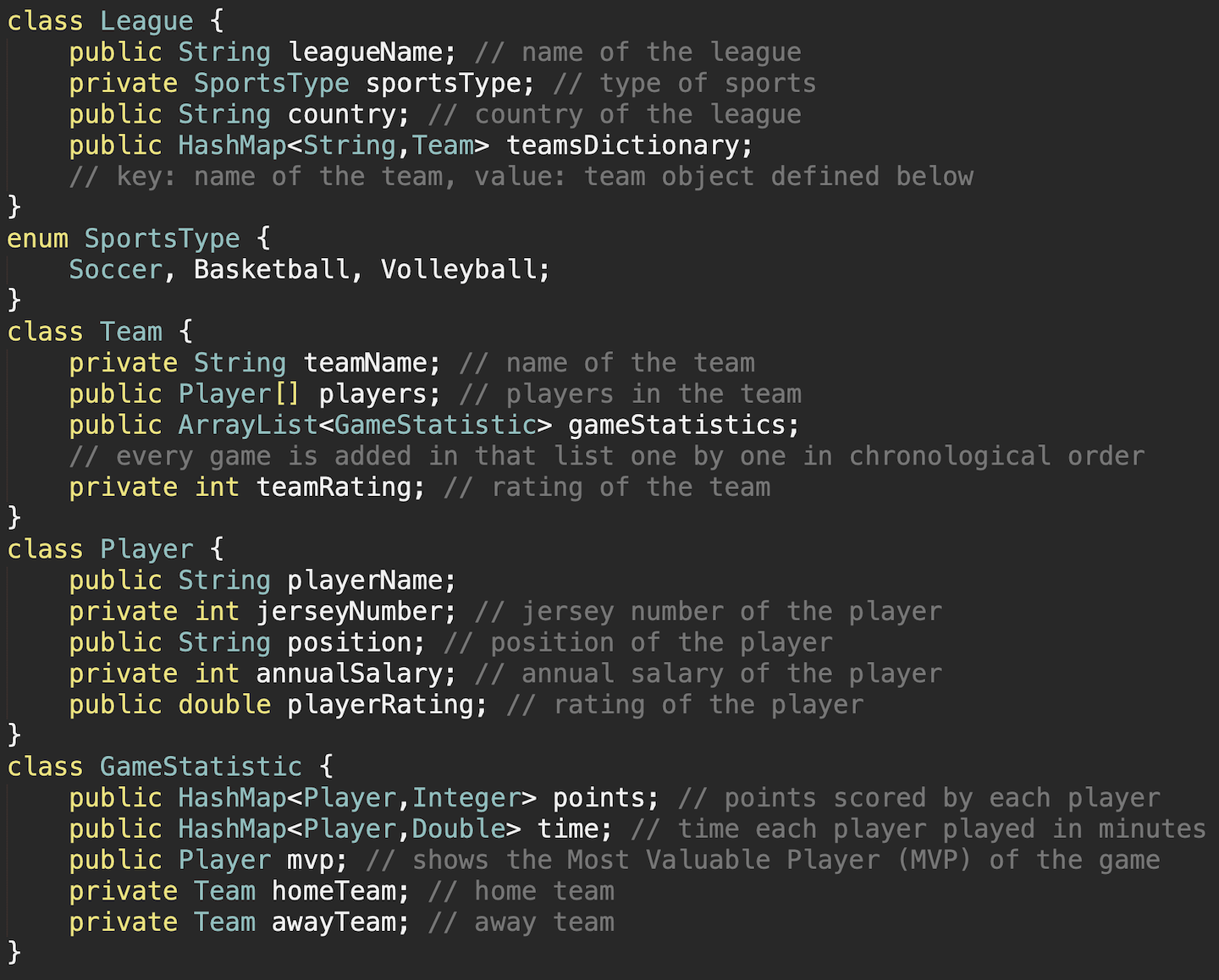1. Provide the code block that performs the following in the main method: Update the name of team “LA Lakers” as “Rich as FB but winners”. How would you change your answer if you knew “LA Lakers” was a “Basketball” team?
2. Provide the code block that performs the following in the main method: Update the name of player “Nick Calathes” with “MVP Nick”.
3. Provide the code block that performs the following in the main method: Print out the number of leagues in France. Print out the number of teams in Germany. Print out the number of Volleyball teams in Italy.
4. I’m about to solve an optimization problem using Gurobi and each League has an associated decision variable, that is continuous, greater than or equal to zero, and less than or equal to 100. I’d like to access that decision variable for League l as X.get(l). Provide the code block that defines and initializes these variables in the main method.
5. Write a method that takes an ArrayList of players as input and returns a subset of the given list of players with maximum total playerRating without exceeding a budget of 10,000,000 with their salary using Gurobi.
6. The player named “James Harden” plays for the team named “New Jersey Nets”. His statistics is missing in the last game of his team and he has the following statistics: 36 points, 29.5 minutes, and he was the MVP of the game. Provide the code block that performs these updates in the main method.
7. The player named “Mesut Ozil” has been transferred from the team named “Arsenal” to the team named “Fenerbahce”. Provide the code block that performs these updates in the main method.
8. Write a mostValuablePlayers method for the Team class, that takes an integer (say n) and returns an ArrayList of players that has become an MVP of the game at least n times.
9. Provide the code block that performs the following in the main method: Update the statistics of the “Basketball” player named “Festus Ezeli”. Note that there are multiple players with the same name, so update the one with jersey number 11. In his last game, his statistics should be updated as follows: 26 points, 19.5 minutes, and he was the MVP of the game. Include any other methods needed and where they should be located.
10. Write a method that takes a GameStatistic object as an input, prints out the name of the MVP of the game, and returns the Player that is the MVP of the game. The same method should also be called with two inputs, a GameStatistic object and a String that denotes the position. In the two input case, the method should still return the Player that is the MVP of the game, but should print out the name of the MVP of the game, only if he/she plays in the position that is input.
11. I’m about to solve an optimization problem for a given type of sports using Gurobi, and each Player of that sport needs an associated decision variable that is continuous, greater than or equal to zero, and less than or equal to 100. In the main method I have the following code:
``````Variables variables = new Variables(); // next I call method initializeAll to initialize decision variables
``````

I’d like to access the decision variable for Player p as variables.getForPlayer(p). Provide the initializeAll method for the Variables class and clearly show how I can call that method from the main method above.

12. Write a method for Team class that adjusts the annualSalary values of all players proportional to their playerRating values. The sum of annual salaries of players in the team should stay the same.
13. Write an updateRandomly method for the League class that randomly picks a Team in the league, and updates the rating of the team with 91. Include any other methods needed and where they should be located.
14. Write a method that takes a GameStatistic object and prints out the names of players in ascending order of points they scored in that game. The print out should be formatted as follows:
``````Player | Points
LeBron James | 36
Thomas Bryant | 25
Anthony Davis | 21